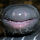# Quadratic Equation Calculator chrome extension

Version: 1.12Find the roots of a quadratic equation!
3.67 Based on 3 user rates

## What is Quadratic Equation Calculator?

Quadratic Equation Calculator is a math solver extension for chrome. also, it is equation calculator extension. it's a free extension , it has 429 active users since released its first version, it earns an average rating of 3.67 from 3 rated user, last update is 2042 days ago.

## What’s new in version 1.12?

```                Are you in high school?
Do you take math?
Do you take physics?
Do you encounter quadratic equations with non-whole number roots?
Are you tired of plugging in coefficients into the quadratic equation?
No longer.
Download this extension and you can find the roots with the click of a button anytime, anywhere.
Watching a Youtube video and you need to solve a quadratic equation?
One Click.
One Quadratic Equation Calculator Extension.            ```

## Technical Features:

• Requirements: Windows Chrome, Mac Chrome
• Latest update: Wednesday, August 16th, 2017
• Author: michaelstrinhs

English.

## Reviews of quadratic equation calculator:

Shleed September 9th, 2020This is espionage, there is not room for two quadratic formula calculators on the chrome web store. Also yours lacks the seizure inducing colors mine possesses in great quantities.